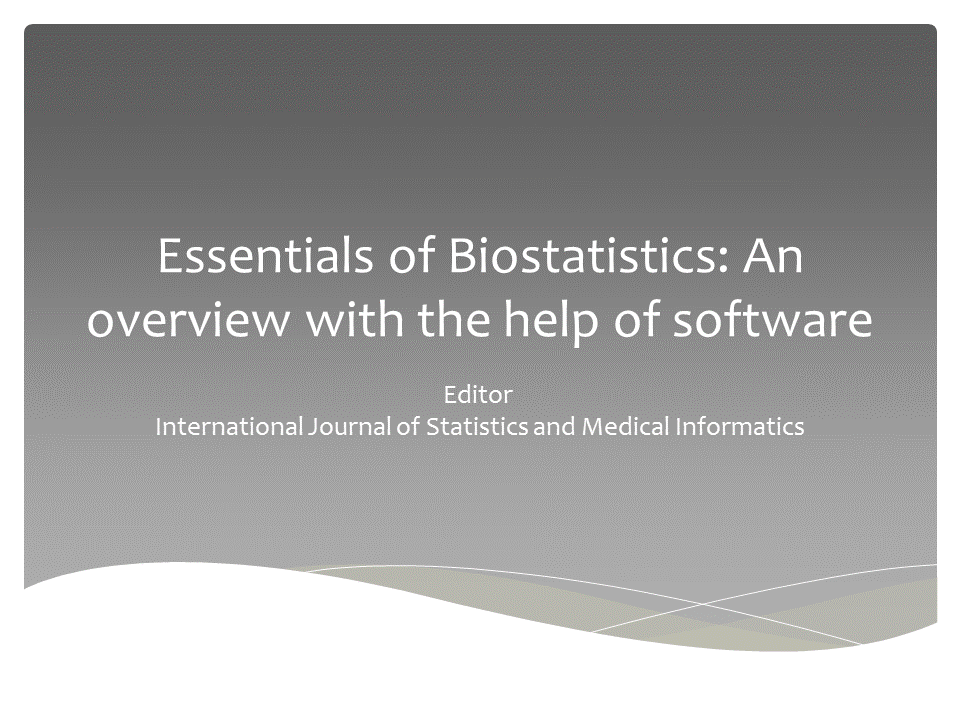# Global Data Science Forum

View Only

## Statistical Models for Data Analysis#### RAUL MARTINWed October 03, 2018 03:54 PM#### swapnali kaduTue January 01, 2019 04:16 AM#### MEKALA REDDYWed February 20, 2019 02:51 AM#### Rocklin DunlapThu January 03, 2019 08:43 AM• #### 1.  Statistical Models for Data Analysis

Posted Wed October 03, 2018 03:54 PM
Hello!

Which are the best statistical models to be used as a MUST when performing data analysis?

------------------------------
RAUL MARTIN
------------------------------

• #### 2.  RE: Statistical Models for Data Analysis

Posted Tue January 01, 2019 04:16 AM

I suggest starting your big data analysis efforts with the following five fundamentals.

### 1. Mean

The arithmetic mean, more commonly known as "the average," is the sum of a list of numbers divided by the number of items on the list. The mean is useful in determining the overall trend of a data set or providing a rapid snapshot of your data. Another advantage of the mean is that it's very easy and quick to calculate.

2. Standard Deviation

The standard deviation, often represented with the Greek letter sigma, is the measure of a spread of data around the mean. A high standard deviation signifies that data is spread more widely from the mean, where a low standard deviation signals that more data align with the mean. In a portfolio of data analysis methods, the standard deviation is useful for quickly determining dispersion of data points.

3. Regression

Regression models the relationships between dependent and explanatory variables, which are usually charted on a scatterplot. The regression line also designates whether those relationships are strong or weak. Regression is commonly taught in high school or college statistics courses with applications for science or business in determining trends over time.

### 4. Sample Size Determination

When measuring a large data set or population, like a workforce, you don't always need to collect information from every member of that population – a sample does the job just as well. The trick is to determine the right size for a sample to be accurate. Using proportion and standard deviation methods, you are able to accurately determine the right sample size you need to make your data collection statistically significant.

5. Hypothesis Testing

Also commonly called testing, hypothesis testing assesses if a certain premise is actually true for your data set or population. In data analysis and statistics, you consider the result of a hypothesis test statistically significant if the results couldn't have happened by random chance. Hypothesis tests are used in everything from science and research to business and economic

------------------------------
------------------------------

• #### 3.  RE: Statistical Models for Data Analysis

Posted Wed February 20, 2019 02:51 AM
• Statistics methods require for DS/DA are: hypothesis testing and summary statistics

------------------------------
MEKALA REDDY
------------------------------

• #### 4.  RE: Statistical Models for Data Analysis

Posted Thu January 03, 2019 08:43 AM
It really depends upon the type of data being analyzed.  Most business analytics can be handled by simple regression or ANOVA analysis.  Medical, military and scientific applications may require far more advanced techniques.
Rock

------------------------------
Rocklin Dunlap
Senior Systems Integrator
Wayne State University
Detroit MI
313-577-1394
------------------------------

• #### 5.  RE: Statistical Models for Data Analysis

Posted Fri February 22, 2019 11:27 PM
The following statistical tools can be used for data analysis
1. Descriptive Statistics
2. Inferential Statistics
3. Analysis Variance
4. Correlation and Regression
5. Bayesian analysis
6. Factor Analysis
7. Discriminant Analysis
8. Cluster Analysis
9. Survival Analysis

Book
Essentials of Biostatistics : An Overview with the help of Software------------------------------
Editor
International Journal Statistics and Medical Informatics
www.ijsmi.com/book.php
------------------------------